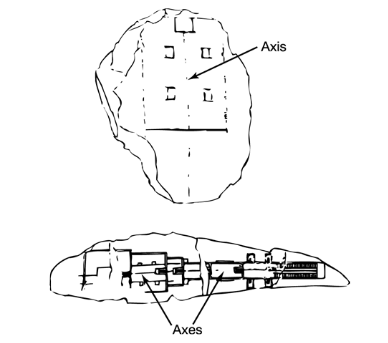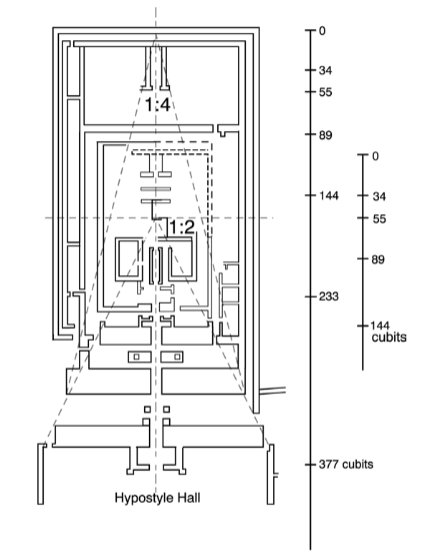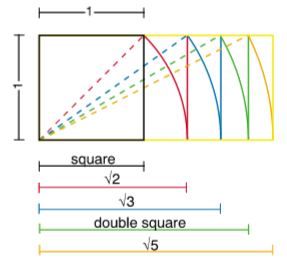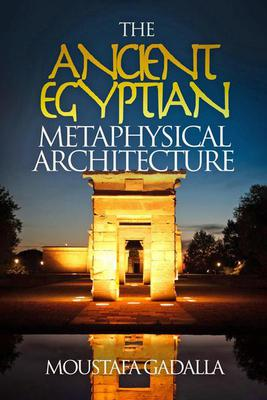# The Harmonic Design Parameters

## The Harmonic Design Parameters

Harmonic design in Ancient Egyptian architecture was achieved through a unification of two systems:

1. Arithmetic (significant numbers).

2. Graphic (square, rectangles, and a few triangles).

The union of the two systems reflects the relationship of the parts to the whole, which is the essence of harmonic design.

This union of arithmetic and graphic design follows the elements described below.

1. The Arithmetic System Consisted Of:

1-a. The Active Axes

An axis is an imaginary and ideal line about which a moving body revolves. In geometry, an axis is equally imaginary—a line without thickness.

The Egyptian temple was regarded as an organic, living unity. It is in constant motion; its intricate alignments and its multiple asymmetries make it oscillate about its axes. This movement takes place within a rhythm given by the “module” or the particular coefficient of the thing or idea to be defined.

Ancient Egyptian architectural design is conspicuous for its strong apparent symmetry around a longitudinal axis. This is the result of the Ancient Egyptian knowledge of cosmic laws. The Egyptian designer reflected such slight cosmic asymmetry by ensuring that elements on either side of the axis are not exactly identical to one another. While most of them are balanced, elements are not symmetrical.Two examples are shown below of  axes defined on Ancient Egyptian drawings.The axis line can be found in a few recovered architectural drawings or sketches on papyri and tablets from various periods. They were, presumably, workmen’s notations, and in spite of their practical purpose, they still feature the axis line drawn in the same conventional way as in modern drawings.

In the buildings themselves, the axis is marked by an engraved line on the stones of the upper course of a foundation slab, such as the case at Luxor Temple.

1-b. Significant Points (Along the Axis)

Significant points were determined along the design axis. These points mark the intersection with transverse axes, the alignment of a central doorway, the position of an altar, the center of the sanctuary, etc. These significant points follow a precise arithmetic progression. In many of the best plans, these significant points are at harmonic distances from one another, and their distances from one end to the other express the figures of the Summation (so-called Fibonacci) Series, 3, 5, 8, 13, 21, 34, 55, 89, 144, 233, 377, 610, . . . The harmonic analysis shows a series of significant points readable from both ends, i.e. if inverted, a system of significant points would also correspond to the Series with the reference point starting at the opposite end of the plan.

High numbers of the Summation Series were crystallized in the Egyptian monuments ever since the Old Kingdom. The design of the pyramid temple of Khafra (Chephren) reaches the figure of 233 cubits in its total length, as measured from the pyramid, with a complete series of TEN significant points.

The Karnak Temple follows the Summation Series’ figures up to 610 cubits, i.e. TWELVE significant points. [See diagrams of both temples in the next chapter].

2. The Graphic System Consisted Of:

2-a. The Telescopic Triangles

The typical Egyptian temple plan increases in width and height from the sanctuary towards the front. This over-all delimitation was based upon a “telescopic system” of design since the Old Kingdom. The increase in width was accomplished by the use of consecutive 1:2, 1:4, and 1:8 triangles from one or more significant point(s). [See diagram of Karnak Temple (partial) below.]The same telescopic configuration applied to the vertical plan, whereby the floor of the temple descended and the roofs ascended outwardly towards the temple’s pylons; as shown in several temples in an earlier chapter of this book.

2-b. The Rectangular Perimeters

The general horizontal and vertical outlines are basically rectangular in shape, for the overall plan as well as its constituent parts. The most common configurations that were used are:

• A simple square, such as that utilized in the Pyramid Temple of Khafra (Chephren) in Giza.
• A double square or 1:2 rectangle, such as the Zoser Complex at Saqqara, the inner enclosure at Karnak, and the festival hall of Twt Homosis III
• Root Rectangles—numerous examples [shown below].• The Neb (Golden) Rectangle, where the “numerical value” of the ratio between the two sides equals 1.618—numerous examples such as in the Pyramid Temple of Khafra in Giza [shown earlier].

## The Vertical Plane

The Ancient Egyptians were masters of the vertical principle as well as the horizontal line. Vertical heights followed the same proportional increase as horizontal widths as additions were made to the front of monuments—an aspect characteristic of the Egyptian temples.

Harmonic proportion was applied by the Ancient Egyptians in all three dimensions, such as:

• The pyramids (square bases and triangle volume).
• The striking case of the King’s Room in Khufu (Cheops) Pyramid, which affords exact relations for the great diagonal in space with respect to the dimension of the side. [See diagram in Chapter 11.]
• Pylons. [See diagram in Chapter 11.]
• Doorways/portals/gates. [See diagram in Chapter 11.]
• Vertical heights followed the same proportional increase as horizontal widths, as additions were made to the front of monuments—an aspect characteristic of the Egyptian temples.

Various applications of harmonic design in Ancient Egyptian works throughout its recovered history—and throughout the land—are found in the next chapter of this book.

[An excerpt from The Ancient Egyptian Metaphysical Architecture by Moustafa Gadalla]
https://egyptianwisdomcenter.org/product/the-ancient-egyptian-metaphysical-architecture/https://egyptianwisdomcenter.org/product/the-ancient-egyptian-metaphysical-architecture/